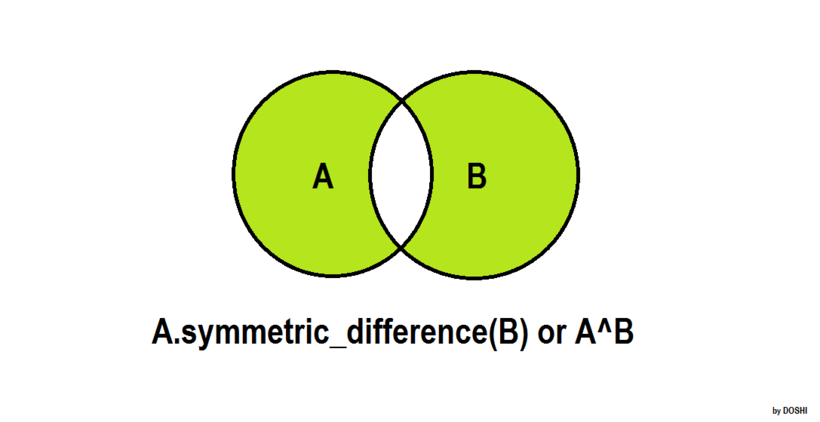## Algorithm

Problem Name: Python - Set .symmetric_difference() Operation

In this HackerRank Functions in PYTHON problem solution,.symmetric_difference()

The .symmetric_difference() operator returns a set with all the elements that are in the set and the iterable but not both.
Sometimes, a `^` operator is used in place of the .symmetric_difference() tool, but it only operates on the set of elements in set.
The set is immutable to the .symmetric_difference() operation (or `^` operation).

``````>>> s = set("Hacker")
>>> print s.symmetric_difference("Rank")
set(['c', 'e', 'H', 'n', 'R', 'r'])

>>> print s.symmetric_difference(set(['R', 'a', 'n', 'k']))
set(['c', 'e', 'H', 'n', 'R', 'r'])

>>> print s.symmetric_difference(['R', 'a', 'n', 'k'])
set(['c', 'e', 'H', 'n', 'R', 'r'])

>>> print s.symmetric_difference(enumerate(['R', 'a', 'n', 'k']))
set(['a', 'c', 'e', 'H', (0, 'R'), 'r', (2, 'n'), 'k', (1, 'a'), (3, 'k')])

>>> print s.symmetric_difference({"Rank":1})
set(['a', 'c', 'e', 'H', 'k', 'Rank', 'r'])

>>> s ^ set("Rank")
set(['c', 'e', 'H', 'n', 'R', 'r'])
``````

Students of District College have subscriptions to English and French newspapers. Some students have subscribed to English only, some have subscribed to French only, and some have subscribed to both newspapers.

You are given two sets of student roll numbers. One set has subscribed to the English newspaper, and one set has subscribed to the French newspaper. Your task is to find the total number of students who have subscribed to either the English or the French newspaper but not both.

Input Format

The first line contains the number of students who have subscribed to the English newspaper.
The second line contains the space separated list of student roll numbers who have subscribed to the English newspaper.
The third line contains the number of students who have subscribed to the French newspaper.
The fourth line contains the space separated list of student roll numbers who have subscribed to the French newspaper.

Constraints

0 < Total number of students in college < 1000

Output Format

Output total number of students who have subscriptions to the English or the French newspaper but not both.

Sample Input

``````9
1 2 3 4 5 6 7 8 9
9
10 1 2 3 11 21 55 6 8
``````

Sample Output

``````8
``````

Explanation

The roll numbers of students who have subscriptions to English or French newspapers but not both are:

4,5,7,9,10,11,21 and 55.

Hence, the total is 8 students.

## Code Examples

### #1 Code Example with Python Programming

```Code - Python Programming```

``````
input()
s = set(input().split())
input()
print(len(s.symmetric_difference(input().split())))
``````
Copy The Code &